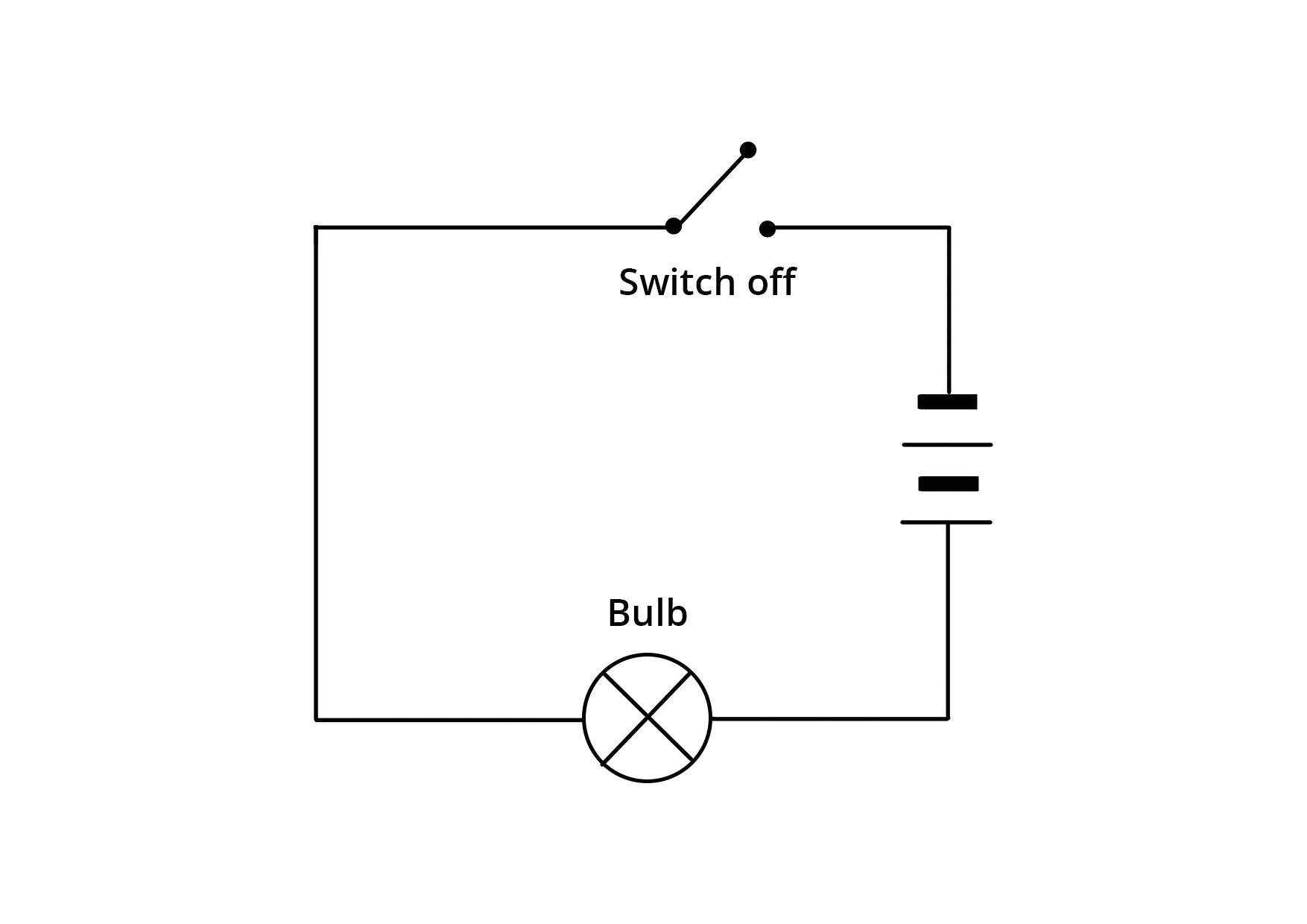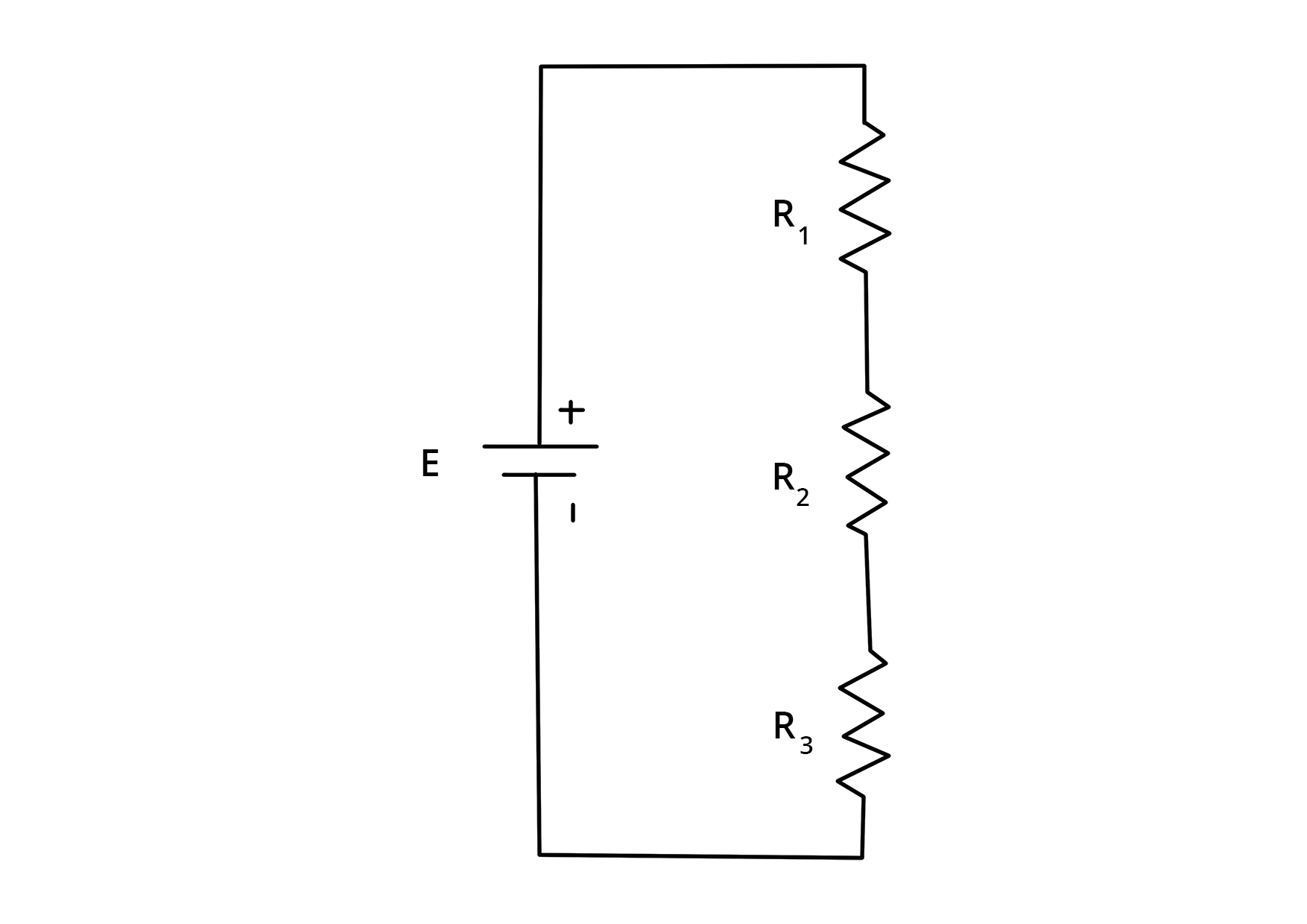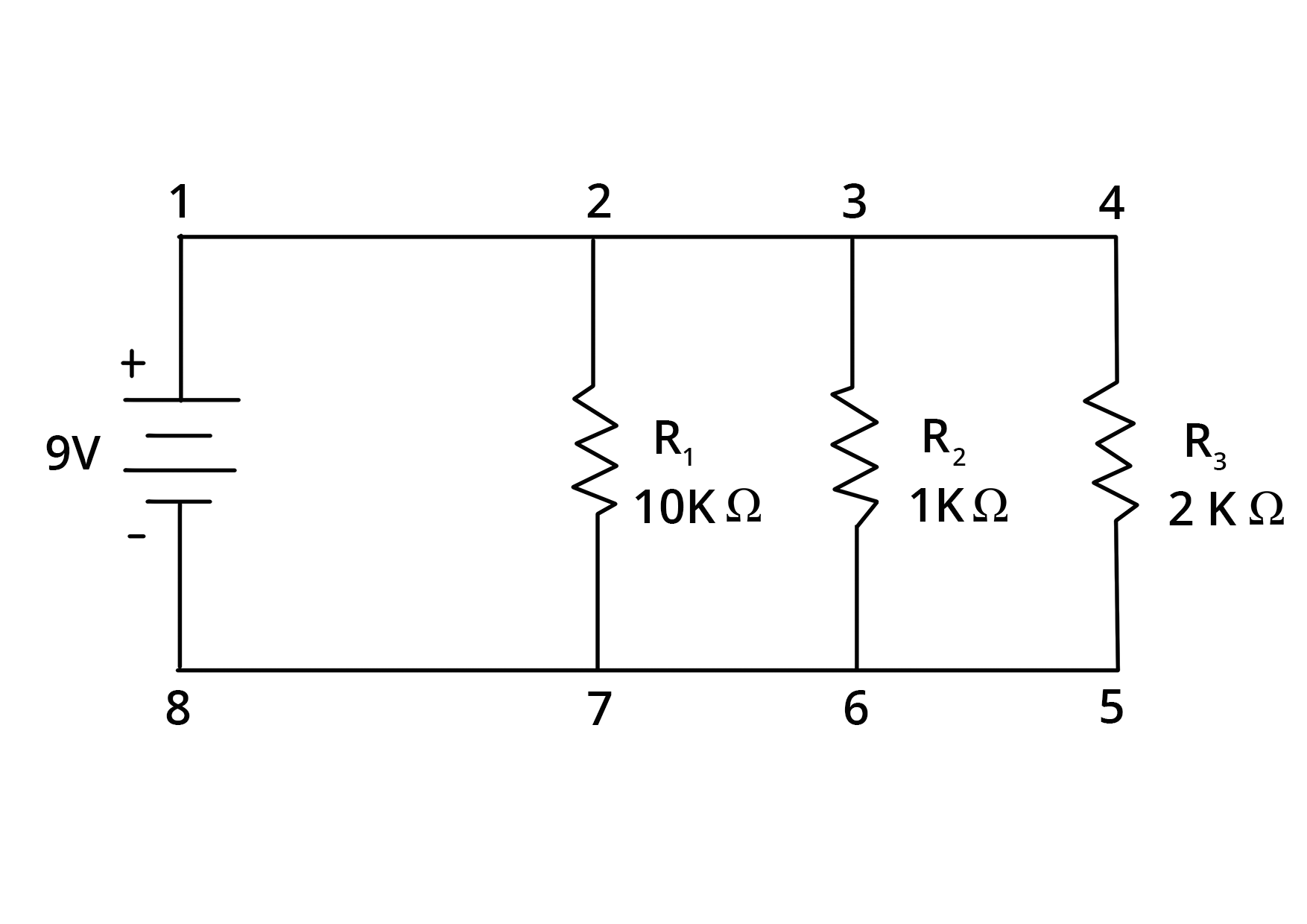• Call Now

1800-102-2727•

# Difference Between Series and Parallel Circuits

Electricity is an important part of modern civilization now. We cannot live without it, not even for a single day. We need to use electricity for most of our tasks in everyday life. A country’s development is mainly based on the improvement of industries, hospitals, and other institutions. So, without electricity, it is impossible to run an industry. It is important to understand the basic concepts and applications of electricity. Some electricity-related components are batteries, switches, keys, resistance, conductors, etc.

In this article, we will discuss the topics related to series and parallel circuits of electricity and their differences as well as similarities. These circuits are used in daily life in various appliances.

## Electrical circuits

An electric circuit is a closed-circuit setup in which a conductor can be connected with positive and negative terminals of a power source. In electrical circuits, there is a switch that can help disconnect the electric flow through the circuits. In order to control the flow, an electric circuit uses a resistor. This resistor can be fitted with a motor, bulb, or other appliances. The connection provided in an electric circuit helps denote its type.## Series connection

A series connection is considered as an electric circuit that contains a connection of circuits that are connected in a series combination. It simply means that one element’s end will be connected to the starting point of the other elements in a circuit.R=R1+R2+R3 The above mentioned is a diagrammatic representation of a series connection. From this design, it can be seen that the same current can flow through all the elements that are connected in the circuit. One thing to remember is that the current is not divided inside the circuit.

## Parallel connection

A parallel connection is another type of electric connection which is a circuit where the starting points of all the components are connected to one common point. Likewise, the finishing points of all the elements are connected to one particular point. In simple terms, the amount of electricity supplied is divided into the total number of branches that emerge from one point in a parallel combination.## Difference between a series circuit and a parallel circuit

One huge difference between the series and parallel circuit is the amount of current that can possibly flow through each of the elements present inside the circuit. As discussed previously, in a series circuit, the flow of current will be in the same amount and it flows through all the elements contained in it. Contrastingly, in parallel circuits, the elements are located in parallel with one another. This is because the circuit splits the flow of current. Hence, the flow of current from the common source is divided into multiple amounts.

Series Parallel
The same amount of current flows through all the elements. The current flowing through each component is capable of combining in order to form the current flow through the source.
In an electrical circuit, components are segregated in a separate line. In an electrical circuit, components are arranged parallel to one other.
When resistors are connected in a series circuit, the voltage throughout each of the resistors is dissimilar even though the flow of line is the same through all of them. When resistors are connected in a parallel circuit, the voltage across each resistor is the same. Even the polarities are the same.
In a series combination, if one component breaks down, the complete circuit will burn out. In parallel combination, other elements will function even if one element breaks down, each has its own independent circuit.
If Vt is the total voltage, then it is equal to V1+V2+V3. If Vt is the total voltage, then it is equal to V1=V2=V3.

The topic Circuit is widely discussed in NCERT Solutions For Class 10 Science Chapter 12, which also talks about  other important concepts like Ohms Law, Semi-Conductors, and Insulators.Talk to our expert
Resend OTP Timer =
By submitting up, I agree to receive all the Whatsapp communication on my registered number and Aakash terms and conditions and privacy policy